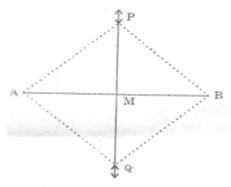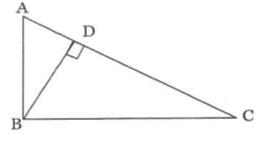# Test: Constructions- 2

## 25 Questions MCQ Test Mathematics (Maths) Class 9 | Test: Constructions- 2

Description
This mock test of Test: Constructions- 2 for Class 9 helps you for every Class 9 entrance exam. This contains 25 Multiple Choice Questions for Class 9 Test: Constructions- 2 (mcq) to study with solutions a complete question bank. The solved questions answers in this Test: Constructions- 2 quiz give you a good mix of easy questions and tough questions. Class 9 students definitely take this Test: Constructions- 2 exercise for a better result in the exam. You can find other Test: Constructions- 2 extra questions, long questions & short questions for Class 9 on EduRev as well by searching above.
QUESTION: 1

Solution:
QUESTION: 2

Solution:
QUESTION: 3

### Radius of a circle is ________ to tangent

Solution:
QUESTION: 4

Two radii of same circle are always :

Solution:
QUESTION: 5

A quadrilateral can be drawn.If the measures of its

Solution: In a rectangle diagonals are equal. Five measurements can determine a quadrilateral uniquely. A quadrilateral can be constructed uniquely if the lengths of its four sides and a diagonal are given.A quadrilateral can be constructed uniquely if its two adjacent sides and three angles are given.
QUESTION: 6

The point in the plane of a triangle which is at equal perpendicular distances from the three sides of the triangle is its

Solution:
QUESTION: 7

The orthocentere of an obtuse angled triangle lies

Solution:
QUESTION: 8

On a ray AB with initial point A, Taking A as centre and some radius, draw an arc of a circle, which intersects AB, say at a point D. Taking D as centre and with the same radius as before, draw an arc intersecting the previously drawn arc, say at a point E. Draw the ray AC passing through E. Then, the measure of ∠CAB is

Solution:
QUESTION: 9

If a 60°angle is bisected twice what will be measure of each angle that is constructed ?

Solution:
QUESTION: 10

In the construction of the perpendicular bisector of a given line segment, as Shown in the figure below ΔPMA ≌ ΔPMB by which congruence criterion?Solution:
QUESTION: 11

An external bisector of an angle measuring 70° will divide the angle into two angles measuring

Solution:
QUESTION: 12

In Δ ABC, which of the following information is needed to construct it if it is known that measure of ∠B = 60 and BC = 6 cm :

Solution:
QUESTION: 13

Which of these triangles are possible to construct by knowing only its altitude?

Solution:
QUESTION: 14

With the help of a ruler and a compass it is not possible to construct an angle of :

Solution:
QUESTION: 15

With the help of a ruler and a compass it is not possible to construct an angle of

Solution:
QUESTION: 16

It is not possible to construct a triangle whose sides are

Solution:
QUESTION: 17

With the help of a ruler and a compass, it is possible to construct an angle of

Solution:
QUESTION: 18

The construction of a triangle ABC with AB = 4 cm and ∠A = 60° is not possible when difference of BC and AC is equal to

Solution:
QUESTION: 19

The construction of a triangle ABC with AB = 6 cm and ∠A = 60° is not possible when sum of BC and CA is equal to

Solution:

Solution :- Given, BC = 6 cm and ∠A=60°

We know that, the construction of a triangle is possible, if sum of two sides is greater than the third side of the triangle i.e.,

AB - BC > AC

⇒  BC + AC > AB

⇒  BC + AC > 6

So, if BC + AC = 7 cm

QUESTION: 20

It is possible to construct a triangle ABC whose sides are

Solution:
QUESTION: 21

The angles ABC and BDC are right angles. If AD = 8 cm and DC = 32 cm the length of BD isSolution:
QUESTION: 22

If D is a mid point of the hypotenuse AC of a right triangle ABC, BD is equal to

Solution:
QUESTION: 23

In Δ ABC if ∠B = ∠C = 300, which of the following is the longest side?

Solution:
QUESTION: 24

In a Δ ABC if ∠A = 50° and ∠B = 70° then the shortest and the largest sides of the triangles are

Solution:
QUESTION: 25

What is the supplementary angle of 108°?

Solution: